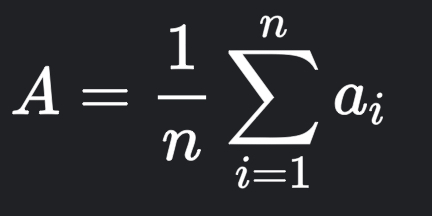# Help me in solving LB01D problem

### My issue

why should we add //2 at the end

### My code

``````# How to find the average of 2 numbers?

A = 15
C = 19
B = (A + C)//2
print(B)

A = 16
C = 18
B = (A + C)//2
print(B)
``````

Learning course: Logic Building in Python
Problem Link: CodeChef: Practical coding for everyone

To find arithmetic mean of N integer, the formula is:A = arithmetic mean
n = number of values
ai = data set values

Or more simplified version is:

Hence, the answer is summing up the two integer A and C and dividing it to 2

Corrected Code

A = 15
C = 19
B = (A + C)//2
print(B)

A = 16
C = 18
B = (A + C)//2
print(B)